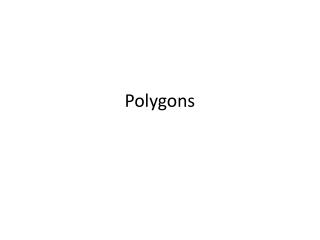DownloadDownload PresentationPolygons

# Polygons

Download Presentation## Polygons

- - - - - - - - - - - - - - - - - - - - - - - - - - - E N D - - - - - - - - - - - - - - - - - - - - - - - - - - -
##### Presentation Transcript

1. Polygons

2. Why a hexagon?

3. Polygon comes from Greek. poly- means "many" -gon means "angle" Polygons 2-dimensional shapes made of straight lines Shape is "closed" (all the lines connect up). Polygon – classified by sides and angles

4. Regular - all angles are equal and all sides are equal complex polygon intersects itself

5. Concave Convex

6. Regular polygons Not regular polygons

7. Concave Octagon Complex Polygon(a "star polygon", in this case, a pentagram) Irregular Hexagon

8. Common polygons

9. Each segment that forms a polygon is a side of the polygon. The common endpoint of two sides is a vertex of the polygon. A segment that connects any two nonconsecutive vertices is a diagonal.

10. Quadrilateral The sum of the angles of a quadrilateral is 360 degrees

11. Parallelogram A four-sided polygon with two pairs of parallel sides. The sum of the angles of a parallelogram is 360 degrees

12. Rectangle A four-sided polygon having all right angles. The sum of the angles of a rectangle is 360 degrees.

13. Square A four-sided polygon having equal-length sides meeting at right angles. The sum of the angles of a square is 360 degrees

14. Rhombus A four-sided polygon having all four sides of equal length. The sum of the angles of a rhombus is 360 degrees.

15. Trapezoid A four-sided polygon having exactly one pair of parallel sides. The two sides that are parallel are called the bases of the trapezoid. The sum of the angles of a trapezoid is 360 degrees.

16. Triangle A three-sided polygon. The sum of the angles of a triangle is 180 degrees.

17. Equilateral Triangle or Equiangular Triangle A triangle having all three sides of equal length. The angles of an equilateral triangle all measure 60 degrees.

18. Isosceles Triangle A triangle having two sides of equal length.

19. Scalene Triangle A triangle having three sides of different lengths.

20. Acute Triangle A triangle having three acute angles.

21. Obtuse Triangle A triangle having an obtuse angle. One of the angles of the triangle measures more than 90 degrees.

22. Right Triangle A triangle having a right angle. One of the angles of the triangle measures 90 degrees. The side opposite the right angle is called the hypotenuse. The two sides that form the right angle are called the legs.

23. Pythagorean Theorem A right triangle has the special property that the sum of the squares of the lengths of the legs equals the square of the length of the hypotenuse.

24. http://www.mathplayground.com/matching_shapes.html

26. Identifying Polygons If it is a polygon, name it by the number of sides. polygon, hexagon

27. Identifying Polygons If it is a polygon, name it by the number of sides. polygon, heptagon

28. Identifying Polygons If it is a polygon, name it by the number of sides. not a polygon

29. Identifying Polygons If it is a polygon, name it by the number of its sides. not a polygon

30. Identifying Polygons If it is a polygon, name it by the number of its sides. polygon, nonagon

31. Identifying Polygons If it is a polygon, name it by the number of its sides. not a polygon

32. A polygon is concave if any part of a diagonal contains points in the exterior of the polygon. If no diagonal contains points in the exterior, then the polygon is convex. A regular polygon is always convex.

33. Classifying Polygons Regular or irregular? Concave or convex? irregular, convex

34. Classifying Polygons Regular or irregular? Concave or convex? irregular, concave

35. Classifying Polygons Regular or irregular? Concave or convex? regular, convex

36. Classifying Polygons Regular or irregular? Concave or convex? regular, convex

37. Classifying Polygons Regular or irregular? Concave or convex? irregular, concave

38. To find the sum of the interior angle measures of a convex polygon, draw all possible diagonals from one vertex of the polygon. This creates a set of triangles. The sum of the angle measures of all the triangles equals the sum of the angle measures of the polygon.

39. By the Triangle Sum Theorem, the sum of the interior angle measures of a triangle is 180°.

40. Example 3A: Finding Interior Angle Measures and Sums in Polygons Find the sum of the interior angle measures of a convex heptagon. (n – 2)180° Polygon  Sum Thm. (7 – 2)180° A heptagon has 7 sides, so substitute 7 for n. 900° Simplify.